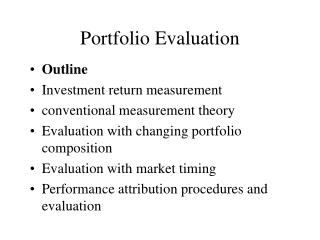DownloadDownload PresentationPortfolio Evaluation

# Portfolio Evaluation

Download Presentation## Portfolio Evaluation

- - - - - - - - - - - - - - - - - - - - - - - - - - - E N D - - - - - - - - - - - - - - - - - - - - - - - - - - -
##### Presentation Transcript

1. Portfolio Evaluation • Outline • Investment return measurement • conventional measurement theory • Evaluation with changing portfolio composition • Evaluation with market timing • Performance attribution procedures and evaluation

2. Measuring Returns • Dollar-weighted returnis the internal rate of return. It is a return equal across a multiperiod. • Time-weighted return is the arithmetic average of each one- period return • Time-weighted return is important for money managers. Because they cannot control cash inflow and outflow for each period, return per period measure is more relevant.

3. Arithmetic Average is simply the average of returns over several periods.Geometric return average is the return over several periods is computed as:(1+rG)=[(1+r1)(1+r2)...(1+rn)]1/n For past returns performance evaluation, the geometric return is a better measure than arithmetic average. For estimating the expected future return, using historic average, arithmetric average is a better as it is an unbiased estimator.

4. Conventional Approaches to Performance Evaluation • Sharpe measure: (rp-rf)/sp is the excess return per unit risk of standard deviation • Treynor measure: (rp-rf)/bp is the excess return per unit systematic risk. • Jensen measure: abnormal returnap =rp - [rf+bp(rm-rf)] • Appraisal ratio: ap/s(ep), which is the alpha (abnormal return) divided by the nonsystematic risk.

5. Evaluations among Different Measures Excess Return Treynor lines . Q . P SML Market 1.0 Beta

6. Treynor measure assumes (1) the portfolio is well-diversified and (2) accurate estimates. Illustration: according to security characteristic line (SCL), a=0.2%, b=1.2,s(e)=2%. The standard error for the “a” is roughly equal to s(a)=s(e)/N1/2 which means for 5% significance, we have the following: t = 1.96 = (a-0)/s(a) = 0.2N0.5/2 N = 384 months (too long to be reliable!)

7. In practice, the portfolio management industry uses a benchment for performance measurement. In academics, other measurements include stochastic dominance method. Frequency g(y) f(x) Return G(y) F(x) 1

8. Changing Portfolio Composition % excess return 27 3 -1 Quarter -9 Mean return (first 4 quarters) =(-1+3-1+3)/4=1% sd =[ (4%+...+4%)/4]0.5=2%

9. Mean of the last 4 quarters: = (-9+27-9+27)/4=9% Sd =[(18%x18%+...]/4]0.5=18% The two years have a Sharpe Measure of 0.5 but the distribution of the return is different. Combination of the two years would yield a mean excess return is 5% and its sd is: [(6%)2+...+(22%)2/8]0.5=13.42% The Sharpe index = 5%/13.42%=0.37(inferior to 0.4 which is the passive strategy and 0.5 individual year) Portfolio mean shift will bias the evaluation performance

10. Market Timing and slope shift of beta • If the proportion between risky asset and riskfree asset is constant, the beta of the entire portfolio remains the same over time as shown below: rp-rf slope=0.6 rm-rf

11. If the portfolio manager shifts funds from the riskfree assets to the risky asset in anticipation of the rise in market return, then we will observe: rp-rf rm-rf Slope of the beta rises

12. That is, there is a regime shift in the regression analysis. To capture the regime shift, we can formulate the several regression models as: (1) rp-rf=a+b(rm-rf)+c(rm-rf)2+ep Hypothesis: c>0 (2) rp-rf=a+b(rm-rf)+c(rm-rf)D+ep where D is a (0,1) dummy - 1 when rm> rf 0 elsewhere. Empirical results show no market timing evidence, i.e., we cannot reject c=0 in both regressions

13. Performance Attribution • Portfolio managers constantly make broad-brush asset market allocation and sector and security allocation within markets • Performance is measured in terms of managed portfolio performance and the benchmark portfolio

14. Benchmark Performance and Excess Return • Component Benchmark Return WeightS&P500 0.6 5.81%Bond Index 0.3 1.45Money Mkt 0.1 0.48 • Benchmark return=0.6x5.81%+0.3x1.45%+0.1x0.48%=3.97% • Managed portfolio excess return=actual return - benchmark=5.34%-3.97%=1.37%

15. Asset Allocation Decisions The performance of the managed fund is due to different proportion of funds allocated as shown: MKT Equity Fixed Inc. TB Actual wt 0.7 0.07 0.23 Benchmark 0.6 0.30 0.10 Excess wt. 0.1 -0.23 0.13 (a) Mkt excess return 1.84 -2.52 -3.49 (b) (5.81-3.97) (1.45-3.97) (0.48-3.97) Contribution 0.184 0.5796 -0.4537 (a x b=) Total contribution =0.1840+0.5796-0.4537=0.3099

16. Sector and Security Selection This analysis captures the super results of the portfolio due to their greater performance: Mkt Equity Fixed Income Return 7.28% 1.89% Index 5.81 1.45 Excess ret 1.47 0.44 (a) Port. wt. 0.7 0.07 (b) Contribution 1.03 0.03 (a x b) Total contribution=1.03+0.03=1.06

17. Portfolio Attribution Summary: Asset allocation 0.31% Sector/security selection 1.06 Total excess return 1.37